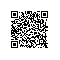# 来一个可能防止恶意采集和爬虫的SH

~~~~~~~~~~~~~~~~~~~~~

$?变量会被赋值为0，反之，赋值为-1。通过判断$?变量的方式确实可以满足我们的要求，但是需要用if判断，要写脚本，比较繁琐，有没有比较方便的命令呢。哈，肯定有的噻。

shell中还提供了快捷操作符: && 和 ||

// 此时存在/tmp/xxxx文件，执行了&&后面的命令
# ls /tmp/xxxx && echo '/tmp/xxxx exits'
/tmp/xxxx
/tmp/xxxx exits

// 此时存在/tmp/xxxx文件，不执行 || 后面的命令
# ls /tmp/xxxx || echo '/tmp/xxxx exits'
/tmp/xxxx

~~~~~~~~~~#/bin/sh

time_inter_minute=50000
log_path="/xxx/xxx/log.log"
last_time=date "-d -${time_inter_minute} minute" '+%d/%b/%G:%H:%M:%S' now_time=date '+%d/%b/%G:%H:%M:%S' now_time_str="[${now_time}"
last_time_str="[${last_time}" echo "start time: "$last_time
echo "end time: " $now_time echo "alert_count: "$alert_count

shell_out=awk -v a=$last_time_str -v b=$now_time_str '$4 > a &&$4 < b' $log_path|awk '{print$1}'|sort |uniq -c | sort -nr|head -10
arra=($shell_out) echo${arra[@]}

for i in "${!arra[@]}"; do if [ expr$i \% 2 -eq 0 ] ;then
if [ ${arra[$i]} -gt $alert_count ] && [${arra[$i+1]} != "xx.xx.xx.xx" ] ; then /sbin/iptables -vnL|grep${arra[$i+1]} && echo${arra[$i+1]} "have added" /sbin/iptables -vnL|grep${arra[$i+1]} || /sbin/iptables -I INPUT -s${arra[\$i+1]} -j DROP
fi
fi
done使用钉钉扫一扫加入圈子
+ 订阅